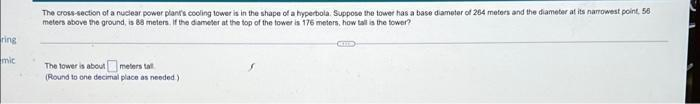Question:

# The cross-section of a nuclear power plants cooling tower is in the shape of a hyperbola. Suppose the tower has a base diameter of 264 meters and the diameter at its narrowest point, 56 meters above tThe cross-section of a nuclear power plants cooling tower is in the shape of a hyperbola. Suppose the tower has a base diameter of 264 meters and the diameter at its narrowest point, 56 meters above the ground, is 88 meters. If the diameter at the top of the tower is 176 meters, how tall is the tower?